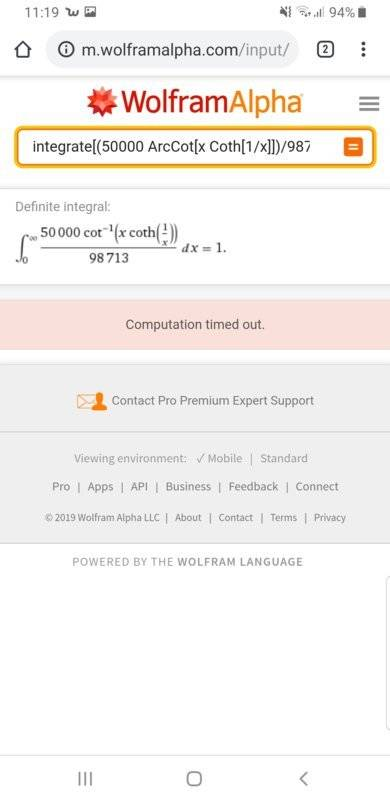# Definite Integral Verification

Saracen Rue
Hi everyone,

I am trying to find the definite integral of a function (see attached image) from 0 to infinity using Wolfram alpha. I'm just looking for some verification on if the integral actual is equal to exactly 1, or if there's some rounding errors going on.

Thank you for your time :)Homework Helper
Gold Member
This is just a guess on my part, but the person who made up the problem may have done a numerical integration, and found the answer to be such that multiplying by the fraction 50,000/98713 made it very close to 1. Perhaps there is a way to evaluate it in closed form, but it is beyond my capabilities.

Saracen Rue
This is just a guess on my part, but the person who made up the problem may have done a numerical integration, and found the answer to be such that multiplying by the fraction 50,000/98713 made it very close to 1. Perhaps there is a way to evaluate it in closed form, but it is beyond my capabilities.

The part that's confusing me is that Wolfram Alpha is usually very good with these type of problems and would provide an answer correct to 100+ decimal places. The fact that it seems to just be rounding it to '1' is quite unusal.

Prehapa the problem is that I don't have Wolfram Alpha Pro which allows for extended computation time. If anyone with Pro does read this, please try computing this question for me and let me know if it provides a different answer or not. Thank you :)

Homework Helper
Dearly Missed

The part that's confusing me is that Wolfram Alpha is usually very good with these type of problems and would provide an answer correct to 100+ decimal places. The fact that it seems to just be rounding it to '1' is quite unusal.

Prehapa the problem is that I don't have Wolfram Alpha Pro which allows for extended computation time. If anyone with Pro does read this, please try computing this question for me and let me know if it provides a different answer or not. Thank you :)

The result is not exactly 1.0000 ... Here is a computation using Maple, set to 30-digit precision.

restart;
k:=50000/98713;

50000
k := -----
98713

> f0:=arccot(x*coth(1/x));

f0 := arccot(x coth(1/x))

> Digits:=30;

Digits := 30

> evalf(Int(f0,x=0..infinity));

1.97425968165579173304278091386

> J0:=%;

J0 := 1.97425968165579173304278091386

> k*J0;

0.999999838752642373873137739639

•